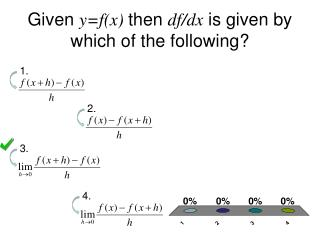DownloadDownload PresentationGiven y=f(x) then df/dx is given by which of the following?

# Given y=f(x) then df/dx is given by which of the following?

Télécharger la présentation## Given y=f(x) then df/dx is given by which of the following?

- - - - - - - - - - - - - - - - - - - - - - - - - - - E N D - - - - - - - - - - - - - - - - - - - - - - - - - - -
##### Presentation Transcript

1. Given y=f(x) then df/dx is given by which of the following? 1 2 3 4 1. 2. 3. 4.

2. equals -2sin2x -sin(2x) 0 -2xsin2x

3. If y=xnthen find dy/dx nxn nxn-1 xn-1 (n-1)xn

4. Find the derivative of f(x)=3x³-½x²+5x+1with respect to x 9x² - 2x + 5 9x³ - x² + 5x + 1 9x² - x + 5 9x³ - x² + 6

5. If -3e3x 3e3x -3e2x -3xe2x

6. f(k)=tan3k, find 3sec3k sec3k 3sec²3k sec²3k

7. = sin(x) -sin(x) cos(x) -cos(x) cosec(x) John Goodband, Coventry University

8. = 1 2 3 4 5 1. 2. 3. 4. 5. John Goodband, Coventry University

9. = 1 2 3 4 5 2. 1. 3. 4. 5. John Goodband, Coventry University

10. Find the derivative of 1 2 3 4 1. with respect to x 2. 3. 4.

11. Find the derivative of z = 2sint – cos2twith respect to t 2cost + sin2t 2cost – sin 2t 2cost + 2sin2t 2cost – 2sin2t

12. If then 1 2 3 4 1. 2. 3. 4. All of the above

13. The derivatie of f(x)+g(x) is The derivative of f(x)-g(x) is If k is constant, the derivative of kf(x) is If y=f(x)g(x) then Which of the following statements are true?

14. equals 2-ecosxsinx +2xcos2x x+ ecosx +2cos2x 2-ecosxsinx +2cos2x Not enough information

15. Find the derivative of y=2xe-xwith respect to x -2xe-x + 2e-x -2xe-x + 2e-x 2xe-x – 2e-x 2xe-x + 2e-x

16. Find the derivative of y=(e2x)6with respect to x 6e2x 12e12x 12xex 12ex

17. = 2xcos(x²) cos(x²) 2xcos(x) x²cos(x²) + 2xsin(x²) John Goodband, Coventry University

18. Which of the following is the quotient rule if ? 1 2 3 4 1. 2. 3. 4.

19. Use the quotient rule to find the derivative of f(x)=x-3cosxwith respect to x 1 2 3 4 1. 2. 3. 4.

20. We know and .Then equals: 5/2 7/2 3

21. Using the chain rule, find the derivative f(x)=(3x²+2)²with respect to x 2(3x² + 2) 12(3x + 2) 12x(3x² + 2) 12x + 4

22. Suppose a runner has a speed of 8 miles per hour, while a cyclist has a speed of 16 miles per hour. Then dV/dt for the cyclist is 2 times greater than dV/dt for the runner. This is explained by: The chain rule The product rule The quotient rule The addition rule

23. The radius of a balloon changes as it deflates. This change in radius with respect to volume is: 1 2 3 4 1. 2. 3. 4. None of these

24. Calculate the second derivative ofy = 4x³ - 2x + x² - 3with respect to x 24x + 2 24x - 2x 12x - 2 12x² - 2 +2x

25. If then find 1 2 3 4 1. 2. 3. 4.

26. If x=h(t) and y=g(t) then 1 2 3 4 1. 2. 3. 4.

27. Find the value of if x=3t2 and y=2t-1. 1 2 3 4 1. 2. 4. 3.

28. If x=h(t) and y=g(t) then 1 2 3 4 2. 3. 4. 1.

29. Find the equation of the tangent line to the curve x=1-3sint, y=2+cost at . 1 2 3 4 1. 2. 3. 4. None of the above

30. Which differentiation rule is needed to differentiate implicit functions? Product rule Chain rule Quotient rule Inverse function rule

31. Find if 3y=xy+siny. 1 2 3 4 1. 2. 3. 4.

32. Find at the point (3,1) on x2+2xy+y2=x. 1 2 3 4 1. 4. 3. 2.